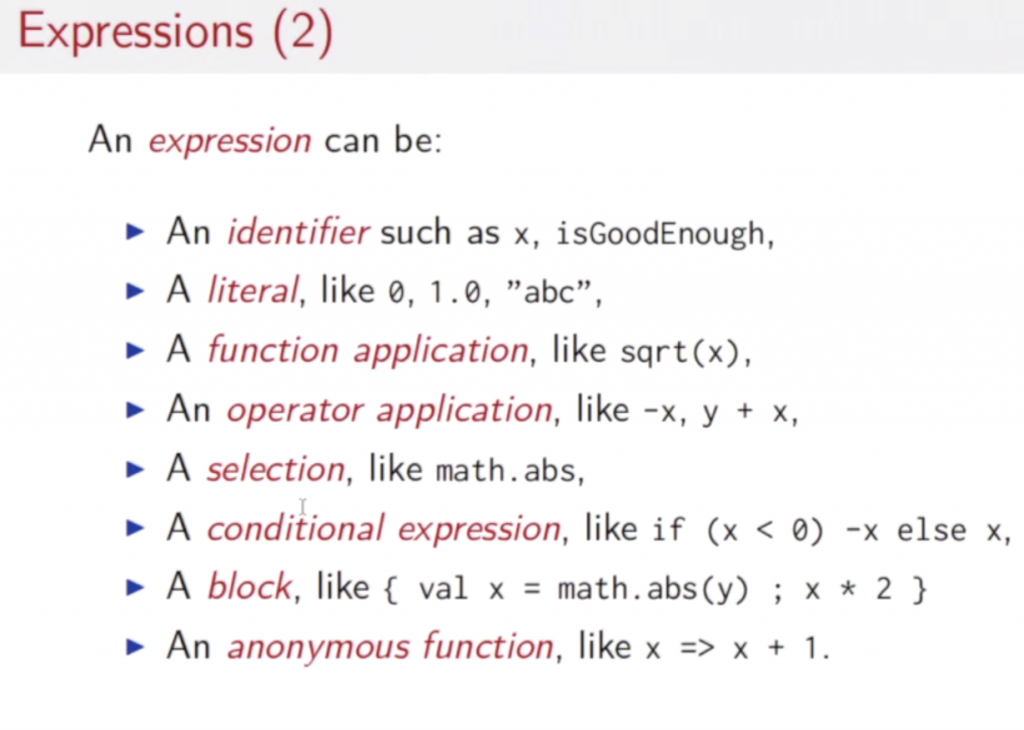# Call by Name vs Call by Value in Scala

This information is based on the coursera course “Functional Programming Principles in Scala” by Martin Odersky.

In Scala expressions* are evaluated by the Scala interpreter following the substitution model. What does this mean? What are expressions, and what means they are evaluated? An expression could be something as simple as “4+3”. Its the combination of some entities. Entities can be primitive or more complex structures. A primitive would be an Integer, a more complex structure could be a function, which is a named expression, so that we can use the name to reference the expression, e.g. “square(3)+square(4)”. “Evaluating an expression” means to reduce it to its value. For example, evaluating the expression 4+3 means that it is reduced to 7. If the expression is (4+3) * pi, you could first reduce 4+3 to 7 and then substitute pi by its value and then finally compute the result. You could also firstly replace pi by its value and then compute 4+3=7. So, the evaluating an expression can be done in various ways. Scala uses the way which is described by the substitution model. Or redundantly spoken: The substitution model is is a model that describes how to do the reduction of an expression to its value. It is formalized in $\lambda$-caluculus. Here is how a function application is evaluated by the Scala interpreter using the substitution model:

1. evaluate all function arguments from left to right
2. replace the function application by its right hand side and at the same time
3. replace the formal parameters of the function by the arguments

Example: You have 2 functions:

def square(x: Double) x*x

def sumOfSquares(x: Double, y: Double) square(x) + square(y)

The below table with the column header “Call by Value” shows how you evaluate the function application sumOfSquares(3, 2+2) using the substitution model.

Now, rule number one above says “evaluate all function arguments from left to right”, so sumOfSquares(3,2+2) in the first step reduces to sumOfSquares(3,4) and then by applying rule 2 (replace the function application by it right hand side and plug in the arguments) we obtain square(3) +square(4). We could also delay the step of the argument evaluation and instead call the function applications right hand side with the unevaluated arguments (if any) and delay the evaluation for later. This strategy is called “Call by Name” and an example is given in the column called “Call by Name” in below table.

 Call by Value comments Call by Value Call by Name Call by Name comments sumOfSquares(3, 2+2) sumOfSquares(3, 2+2) replace all arguments from left to right sumOfSquares(3,4) square(3) + square(2+2) The evaluation of the function arguments is delayed, instead the right hand side substitution is done with the arguements as given replace the func app by its right hand side and plug in the arguments square(3)+square(4) 3*3 + square(2+2) evaluate replace the func app by its right hand side and plug in the arguments from left to right 3*3 + square(4) 9 + square(2+2) evaluate evaluate (from left to right) 9 + square(4) 9+ (2+2) * (2+2) The evaluation of the function arguments is delayed, instead the right hand side substitution is done with the arguements as given replace the func app by its right hand side and plug in the arguments 9 + 4*4 9+4*(2+2) evaluate evaluate 9 + 16 9+4*4 evaluate evaluate 25 9+16 evaluate evaluate 25 evaluate
• The substitution model can only be applied to expressions without side-effects. A side effect is an expression like a++. This cannot be evaluated by a simple rewrite – you would need a store to hold the current value of a.
• Not every expression can be reduced in a finite number of steps. If you have an expression that is such that when substituted calls itself you have created a substitution loop. e.g. def x = x would result in the substitution of x by x and so on.

It is more advantageous (requiring less evalutaion steps than Call by Name) to use Call by Value if the function parameters are used frequently. E.g. calling  def someFun(x: Int, y: Int) x+x +x*y + y*y with someFun(3+4,2+3) would evaluate to someFun(7,2+3)-> somFun(7,5) -> 7+7+7*5+7*7. If you used Call by Name the evaluation would be: someFun(3+4,2+3)-> (3+4)+(3+4)+(3+4)*(2+3)+(2+2)*(2+3). So in the latter case you would need more evaluation steps. But if a function parameter might not be used at all, Call by Value would be quicker. E.g. def someOtherFun(x: Int, y: Int) x+x (note x+x, not x+y!) called like that: someOtherFun(3,4+8) -> 3+3->6. Here, the expression 4+8 is never used. Call by Name would have evaluted the function call as follows: someOtherFun(3,4+8) -> someOtherFun(3,12) -> 3+3->6.

In Scala we can enforce to use Call by Value if we place the sign “=> ” in front of the function parameter type, like this:

def someFun( x: => Int, y: => Int) x+x +x*y + y*y

Now the evaluation would be: someFun(3,4+8)-> 3+3 + 3*(4+8)+(4+8)*(4+8)

*Expressions can be (taken from Martin Oderskys “Functional Programming in Scala” ) coursera course week 2):